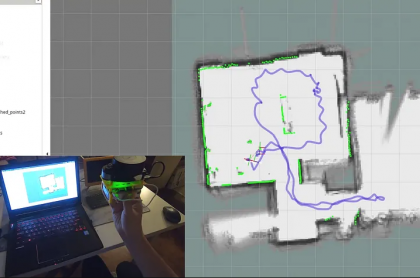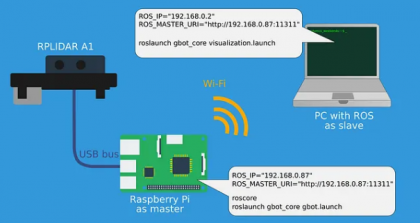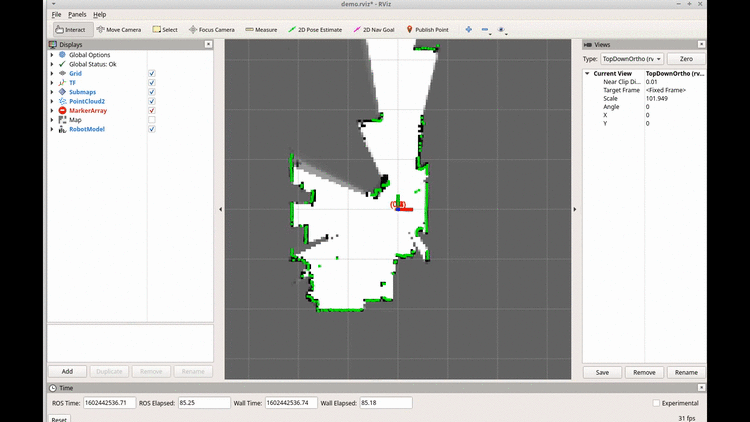# 使用 Google Cartographer 和 RPLidar 与 Raspberry Pi 进行机器人SLAM建图Cartographer 是一个提供实时 SLAM（同时定位和映射）的系统。换句话说，使用来自激光雷达和其他传感器的信息，Cartographer 可以构建环境地图并显示机器人与地图相关的位置。Cartographer 可以构建二维地图和 3D。但对于 3D 地图，需要点云作为源。我们将使用 RPLIDAR a1m8 作为主要传感器。该传感器提供 360 度距离测量作为细线 — Laserscan。Cartorgapher 支持多种激光雷达、IMU 和其他

— cartographer_ros您可以从源代码构建它或从 ROS 存储库获取。对于第一次尝试，我会推荐第二个选项。

## 解释软件部分## 树莓派

sudo apt install ros-melodic-cartographer-ros ros-旋律-rplidar-ros

git   clone   https://github.com/Andrew-rw/gbot_core.git

configuration_files目录中，您可以找到 Cartographer 的配置：

```
include "map_builder.lua"
include "trajectory_builder.lua"
options = {
map_builder = MAP_BUILDER,
trajectory_builder = TRAJECTORY_BUILDER,
map_frame = "map",
odom_frame = "odom",
provide_odom_frame = true,
publish_frame_projected_to_2d = true,
use_odometry = false,
use_nav_sat = false,
use_landmarks = false,
num_laser_scans = 1,
num_multi_echo_laser_scans = 0,
num_subdivisions_per_laser_scan = 1,
num_point_clouds = 0,
lookup_transform_timeout_sec = 0.2,
submap_publish_period_sec = 0.3,
pose_publish_period_sec = 5e-3,
trajectory_publish_period_sec = 30e-3,
rangefinder_sampling_ratio = 1.,
odometry_sampling_ratio = 1.,
fixed_frame_pose_sampling_ratio = 1.,
imu_sampling_ratio = 1.,
landmarks_sampling_ratio = 1.,
}
MAP_BUILDER.use_trajectory_builder_2d = true
TRAJECTORY_BUILDER_2D.min_range = 0.5
TRAJECTORY_BUILDER_2D.max_range = 8.
TRAJECTORY_BUILDER_2D.missing_data_ray_length = 8.5
TRAJECTORY_BUILDER_2D.use_imu_data = false
TRAJECTORY_BUILDER_2D.use_online_correlative_scan_matching = true
TRAJECTORY_BUILDER_2D.real_time_correlative_scan_matcher.linear_search_window = 0.1
TRAJECTORY_BUILDER_2D.real_time_correlative_scan_matcher.translation_delta_cost_weight = 10.
TRAJECTORY_BUILDER_2D.real_time_correlative_scan_matcher.rotation_delta_cost_weight = 1e-1
-- for current lidar only 1 is good value
TRAJECTORY_BUILDER_2D.num_accumulated_range_data = 1
POSE_GRAPH.constraint_builder.min_score = 0.65
POSE_GRAPH.constraint_builder.global_localization_min_score = 0.65
POSE_GRAPH.optimization_problem.huber_scale = 1e2
POSE_GRAPH.optimize_every_n_nodes = 35
return options
```

TRAJECTORY_BUILDER_2D.use_imu_data = false表示我们不会使用 IMU 并且只计算 Laserscan 点匹配算法。您可以在 Cartographer 文档中

```
<material name="orange">
<color rgba="1.0 0.5 0.2 1" />
</material>
<material name="gray">
<color rgba="0.2 0.2 0.2 1" />
</material>
<visual>
<origin xyz="0 0 0" />
<geometry>
</geometry>
<material name="gray" />
</visual>
<visual>
<origin xyz="0.01 0 0.015" />
<geometry>
<box size="0.11 0.065 0.052" />
</geometry>
<material name="orange" />
</visual>
<joint name="laser_joint" type="fixed">
<origin rpy="0 0 3.1415926" xyz="0 0 0.05" />
</joint>
</robot>
```

```
<launch>
<!-- Load robot description and start state publisher-->
<node name="robot_state_publisher" pkg="robot_state_publisher" type="robot_state_publisher" />

<!-- Start RPLIDAR sensor node which provides LaserScan data  -->
<node name="rplidarNode" pkg="rplidar_ros" type="rplidarNode" output="screen">
<param name="serial_port" type="string" value="/dev/ttyUSB0"/>
<param name="serial_baudrate" type="int" value="115200"/>
<param name="frame_id" type="string" value="laser"/>
<param name="inverted" type="bool" value="false"/>
<param name="angle_compensate" type="bool" value="true"/>
</node>

<!-- Start Google Cartographer node with custom configuration file-->
<node name="cartographer_node" pkg="cartographer_ros" type="cartographer_node" args="
-configuration_directory
\$(find gbot_core)/configuration_files
-configuration_basename gbot_lidar_2d.lua" output="screen">
</node>
<!-- Additional node which converts Cartographer map into ROS occupancy grid map. Not used and can be skipped in this case -->
<node name="cartographer_occupancy_grid_node" pkg="cartographer_ros" type="cartographer_occupancy_grid_node" args="-resolution 0.05" />

</launch>

```

visualization.launch的结构更简单。它的目的是用准备好的视图配置启动rviz

```
<launch>
<!-- Start RViz with custom view -->
<node pkg="rviz" type="rviz" name="show_rviz" args="-d \$(find gbot_core)/rviz/demo.rviz"/>
</launch>
```

## 笔记本电脑

`sudo apt install  ros-melodic-cartographer-rviz`

## Cartographer测试

roslaunch gbot_core gbot.launchhttps://miro.medium.com/v2/resize:fit:720/1*8ImKqBVghzGgsvGWvz6foA.gif## 结论

Cartographer 可以提供质量不错的 2D 里程计，只使用低成本的 360 度 LDS 和相当低的数据速率（5-7 赫兹）。
IMU 和额外的里程计源（例如轮式平台里程计或视觉里程计）可以提高大环境区域的地图质量。但对于大约 50-60 平方米的室内地图，它们并不那么重要——Cartographer 的内部闭环算法能够保持这种小地图的一致性。